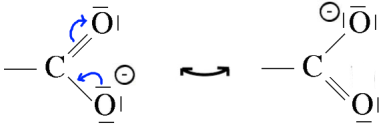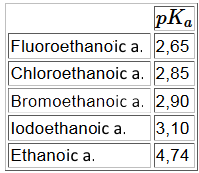Search:

# The acidity of carboxylic acids

## The facts

- The $pH$ of an $1\;M$ aqueous solution of ethanoic acid is $2.4$, that of a solution of a $1\;M$ hydrochloric acid (fully dissociated) is $0$: The dissociation of ethanoic acid is weak! - $1\;mol$ ethanoic is neutralized by only $1\;mol$ of sodium hydroxide: Only one of the four atoms $H$ of $CH_3COOH$ is acidic!

## Dissociation

Admittedly carboxylic acids dissociate following (Arrhenius theory):

$R-COOH$ $\leftrightarrows$ $R-COO^-$ $+$ $H^+$

In fact, the hydrogen ions $H^+$ resulting from this dissociation are immediately taken up from water to form hydronium ions (Broenstedt theory):

$R-COOH$ $+$ $H_2O$ $\leftrightarrows$ $R-COO^-$ $+$ $H_3O^+$

## Equations

The equations of these acidic substances reactions are therefore presented in the context - either in nonionic form, e.g. $CH_3COOH+NaOH\rightarrow CH_3COONa+H_2O$ - either in simplified ionic form, e.g. $CH_3COO^-$ $+$ $H^+$ $+$ $Na^+$ $+$ $OH^-$ $\rightarrow$ $CH_3COO^-$ $+$ $Na^+$ $+$ $H_2O$ - either in complete ionic form, e.g. $CH_3COO^-$ $+$ $H_3O^+$ $+$ $Na^+$ $+$ $OH^-$ $\rightarrow$ $CH_3COO^-$ $+$ $Na^+$ $+$ $2H_2O$

## The acidity of the carboxyl group

Three causes can be invoked to explain the acidity of the carboxyl group: 1) The -I effect of the carbonyl group:This effect enhances the polarization of the $OH$ bond, which makes it easier for $H^+$ to leave. 2) The mesomerism of the carboxyl group:The appearance of a positive charge on the oxygen in a contributive structure reinforces the polarization of $OH$ binding, which makes it easier which makes it easier for $H^+$ to leave. 3) The mesomerism of the carboxylate ion:Mesomerism this is perfect because both contributive structures have the same probability. The carboxylate ion is stabilized considerably by mesomerism, which shifts the equilibrium dissociation to the acid side, so to the departure of $H^+$ is much easier.

## The influence of the hydrocarbon chain$R$ chains that exerce a $+ I$ effect on the carboxyl group decrease the polarity of the $OH$ bond make it more difficult for $H^+$ to leave, for example:Reminders: More the $pK_a$ is large, the less acid is the substance. The hydrocarbon chain has a stronger $+I$ effect especially as it is long or branched.

$R$ chains that have a $-I$ effect on the carboxyl group increase the polarity of the $OH$ bond and make the start of $H^+$ easier, for example:Reminders: E.n.(F)>E.n.(Cl)>E.n.(Br)>E.n.(I)>Effect of the number of electronegative atomsEffect of the distance of electronegative atoms